# Multiple piecewise constant regression

An algorithm for constructing a piecewise constant dependence of the variable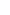from the weighted sum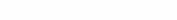minimizing the sum of squared deviationsfrom the average values ​​on the ranges of the value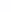.

## Motivation

The problem of restoring the dependence of a certain value on a number of features is common in data analysis. Piecewise constant regression will be useful if it is required to identify the characteristic ranges of changes in the integral feature (weighted sum of features) in order to consider separately the points belonging to these ranges. Thus, having received information about the distribution of points within each of them and examining the dependence on other factors, we can draw conclusions about the segmented data.

## Formal staging

Input data – set of points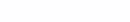and number of ranges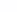.

Define the objective function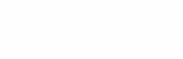depending on the ranges of change in the valuewhere in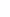-th range includes points with indices from the set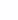And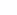is the arithmetic mean of the values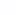Where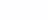.

1. It is required to optimally split the points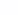into ranges and find a partition such that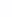minimum.

2. It is required to find such weight coefficients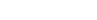for which the indicated minimum, found for given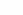will be the smallest.

## Algorithm

The first problem is solved by the dynamic programming method. Let’s order the points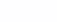ascending coordinates. Select subtasks with parameters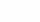– split points with indices from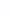beforeonranges. Knowing the solution to the subproblemtrying to improve the solution of subproblems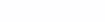For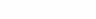filling in the matrices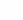– minimum objective function,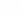– the size of the last range in the optimal partition. Finally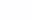will be equal to the minimum of the objective function, and the partition itself can be restored from the values.

The second problem is discrete, and since the value of the objective function depends only on the order of the pointsthen depending on the argumentsthe objective function is piecewise constant, so the standard non-linear optimizers are not applicable here.

For selection of coefficientsthe following iterative method is proposed. The coefficients are chosen as the initial approximationcalculated from multiple linear regression. Then, at each iteration of the algorithm, we will calculate the optimal partition for the found weight coefficients, after which we fix the sizes of the partition ranges and optimize the weight coefficients for the given range sizes.

Thus, we come to the next subtask:

1. It is required to find the coefficientsfor which the objective function will be minimal among all partitions into ranges of a given size.

This problem is proposed to be solved by the fastest coordinate-wise descent in each variable. So let’s define the functions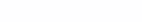. Need to find this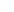at which the value of the objective function(given range sizes) computed for points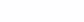will be the smallest.

The last problem belongs to computational geometry and can be solved in different ways. The naive method is to enumerate all the intersection points of the function graphs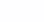And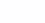and calculating for each point the value of the objective functionfollowed by selection of the best. The complexity of the algorithm will be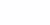.

The other way is similar to the convex hull gift wrapping algorithm. This method plots the order statistics of the setsascending. We are interested in the whole set of events when, with a gradual increasethe contents of the ranges will change due to the permutation of a point on the border of the ranges with a point from the neighboring range. Since there are no more such events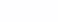then the complexity of the algorithm will be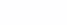.

And finally, it is possible, as in Graham’s algorithm, to arrange all points in ascending orderand add points one by one, each time updating the solution to the subproblem of plotting order statistics depending onfor the points already considered. Because the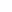order statistics can be constructed in timethen finding the minimum of the objective function will take the same time.

Thus, to optimize the objective function valuefor given sizes of ranges by coefficientswe will optimize in turn the coefficient in each term in the set of points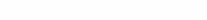taking the remaining terms in this sum as a free term.